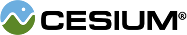#Intersections2D

#### Intersections2D() Core/Intersections2D.js 17

Contains functions for operating on 2D triangles.

### Methods

#### staticCesium.Intersections2D.clipTriangleAtAxisAlignedThreshold(threshold, keepAbove, u0, u1, u2, result) → Array.<Number> Core/Intersections2D.js 45

Splits a 2D triangle at given axis-aligned threshold value and returns the resulting polygon on a given side of the threshold. The resulting polygon may have 0, 1, 2, 3, or 4 vertices.
Name Type Description
`threshold` Number The threshold coordinate value at which to clip the triangle.
`keepAbove` Boolean true to keep the portion of the triangle above the threshold, or false to keep the portion below.
`u0` Number The coordinate of the first vertex in the triangle, in counter-clockwise order.
`u1` Number The coordinate of the second vertex in the triangle, in counter-clockwise order.
`u2` Number The coordinate of the third vertex in the triangle, in counter-clockwise order.
`result` Array.<Number> optional The array into which to copy the result. If this parameter is not supplied, a new array is constructed and returned.
##### Returns:
The polygon that results after the clip, specified as a list of vertices. The vertices are specified in counter-clockwise order. Each vertex is either an index from the existing list (identified as a 0, 1, or 2) or -1 indicating a new vertex not in the original triangle. For new vertices, the -1 is followed by three additional numbers: the index of each of the two original vertices forming the line segment that the new vertex lies on, and the fraction of the distance from the first vertex to the second one.
##### Example:
``````var result = Cesium.Intersections2D.clipTriangleAtAxisAlignedThreshold(0.5, false, 0.2, 0.6, 0.4);
// result === [2, 0, -1, 1, 0, 0.25, -1, 1, 2, 0.5]``````

#### staticCesium.Intersections2D.computeBarycentricCoordinates(x, y, x1, y1, x2, y2, x3, y3, result) → Cartesian3 Core/Intersections2D.js 234

Compute the barycentric coordinates of a 2D position within a 2D triangle.
Name Type Description
`x` Number The x coordinate of the position for which to find the barycentric coordinates.
`y` Number The y coordinate of the position for which to find the barycentric coordinates.
`x1` Number The x coordinate of the triangle's first vertex.
`y1` Number The y coordinate of the triangle's first vertex.
`x2` Number The x coordinate of the triangle's second vertex.
`y2` Number The y coordinate of the triangle's second vertex.
`x3` Number The x coordinate of the triangle's third vertex.
`y3` Number The y coordinate of the triangle's third vertex.
`result` Cartesian3 optional The instance into to which to copy the result. If this parameter is undefined, a new instance is created and returned.
##### Returns:
The barycentric coordinates of the position within the triangle.
##### Example:
``````var result = Cesium.Intersections2D.computeBarycentricCoordinates(0.0, 0.0, 0.0, 1.0, -1, -0.5, 1, -0.5);
// result === new Cesium.Cartesian3(1.0 / 3.0, 1.0 / 3.0, 1.0 / 3.0);``````